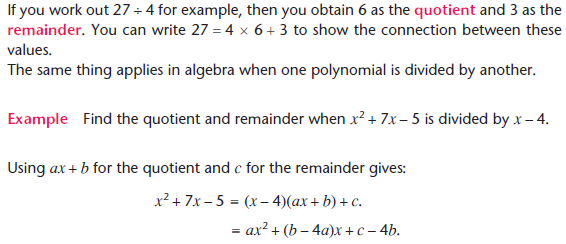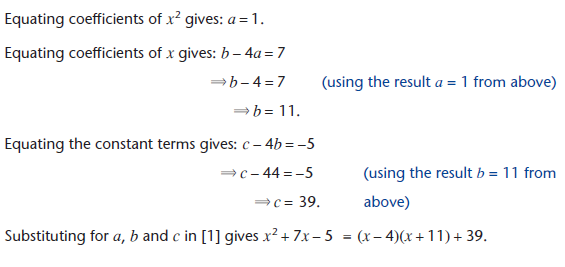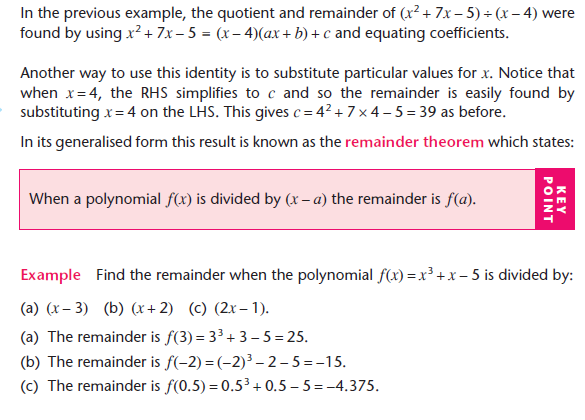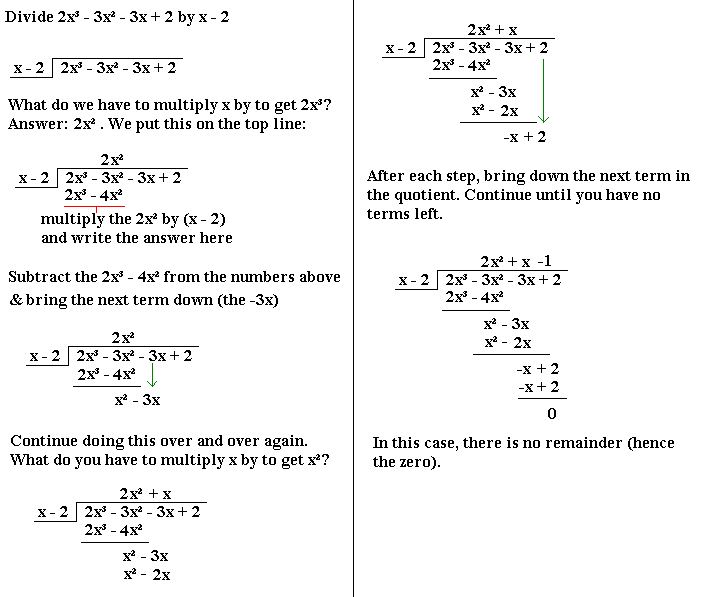## Algebraic Long DivisionAlgebraic long division is very similar to traditional long division (which you may have come across earlier in your education). The easiest way to explain it is to work through an example.

ExampleNB:
If the polynomial/ expression that you are dividing has a term in x missing, add such a term by placing a zero in front of it. For example, if you are dividing x³ + x - 4 by something, rewrite it as x³ + 0x² + x - 4 .

With algebraic long division, practise makes perfect- the best way to learn how to do them properly is to do loads of examples until you get them right every time.

The Factor Theorem

This states:

• If (x – a) is a factor of the polynomial f(x), then f(a) = 0

In other words, if a polynomial f(x) can be divided by (x - a) without a remainder, then x = a is a root of f(x) (so f(a) = 0).

In the above worked example, f(2) = 0. This means that (x - 2) is a factor of the equation.

The factor theorem is important because it can be an easy way of finding factors that would otherwise be difficult to find.

Example

Factorise x3 - 7x - 6.

Note that if we replace x by -1, then we get zero. If you notice or have been told this, then you know immediately, by the factor theorem, that one of the factors is (x + 1). Now we can divide x3 -7x - 6 by (x + 1) to find the other factors. If you carry out the division, you will get x2 - x - 6 . This is easy to factorise, the answer being (x - 3)(x + 2) .

So x3 -7x - 6 = (x + 1)(x - 3)(x + 2)

The Remainder Theorem

When dividing one algebraic expression by another, more often than not there will be a remainder. It is often useful to know what this remainder is and it can often be calculated without going through the process of dividing. The rule is:

• If a polynomial f(x) is divided by ax - b, the remainder is f(b/a)

In the above example, 2x³ - 3x² - 3x + 2 was divided by x - 2.

Let f(x) = 2x³ - 3x² - 3x + 2 . In this case, a = 1, b = 2. The remainder is therefore f(2) = 2×2³ - 3×2² - 3×2 + 2 = 0, as we saw when we divided the whole thing out.

Terminology

The quotient is what you are given after dividing. So, if p(x) is the original polynomial, then

p(x) = q(x)s(x) + r(x), where q(x) is the quotient, s(x) is what you are dividing by and r(x) is the remainder.